When propane is reacted with conc. ${\mathrm{HNO}}_{3}$ at $400-500°\mathrm{C}$ the number of nitro alkanes obtained are

1. 1                              2. 2

3. 3                              4. 4

Concept Questions :-

Alkanes, Alkenes and Alkynes - Nomenclature and Isomerism
High Yielding Test Series + Question Bank - NEET 2020

Difficulty Level:

$\mathrm{CH}\equiv \mathrm{CH}\stackrel{{\mathrm{O}}_{3}/\mathrm{NaOH}}{\to }$X$\stackrel{\mathrm{Zn}/{\mathrm{CH}}_{3}\mathrm{COOH}}{\to }$Y

Y is:

1.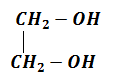2. ${\mathrm{CH}}_{3}{\mathrm{CH}}_{2}\mathrm{OH}$

3. ${\mathrm{CH}}_{3}\mathrm{COOH}$                       4. ${\mathrm{CH}}_{3}\mathrm{OH}$

Concept Questions :-

Alkanes, Alkenes and Alkynes - Chemical Properties
High Yielding Test Series + Question Bank - NEET 2020

Difficulty Level:

Which alkene has smallest heat of hydrogenation?

1. ${\mathrm{CH}}_{3}-\mathrm{CH}=\mathrm{CH}-{\mathrm{CH}}_{3}$

2. ${\left({\mathrm{CH}}_{3}\right)}_{2}\mathrm{C}=\mathrm{CH}-{\mathrm{CH}}_{3}$

3. ${\mathrm{CH}}_{2}=\mathrm{CH}-{\mathrm{CH}}_{2}-{\mathrm{CH}}_{3}$

4. ${\mathrm{CH}}_{3}-\mathrm{CH}=\mathrm{CH}-\mathrm{CH}{\left({\mathrm{CH}}_{3}\right)}_{2}$

Concept Questions :-

Alkanes, Alkenes and Alkynes - Chemical Properties
High Yielding Test Series + Question Bank - NEET 2020

Difficulty Level:

$\left({\mathrm{CH}}_{3}{\right)}_{3}\mathrm{CH}$ + ${\mathrm{Cl}}_{2}\stackrel{\mathrm{hv}}{\to }$ A(Major), the compound 'A' is

(1 mole)

1. $\left({\mathrm{CH}}_{3}{\right)}_{2}\mathrm{CH}-{\mathrm{CH}}_{2}-\mathrm{Cl}$

2. $\left({\mathrm{CH}}_{3}{\right)}_{3}\mathrm{C}-\mathrm{Cl}$

3. Equimolar mixture of (1) and (2)

4. No reaction

Concept Questions :-

Alkanes, Alkenes and Alkynes - Chemical Properties
High Yielding Test Series + Question Bank - NEET 2020

Difficulty Level:

Which of the following alkane will give maximum number of isomers when monochloro substituted product is obtained

(1)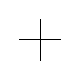(2)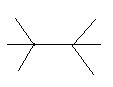(3) ${\mathrm{CH}}_{4}$                                        (4)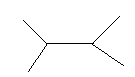Concept Questions :-

Alkanes, Alkenes and Alkynes - Nomenclature and Isomerism
High Yielding Test Series + Question Bank - NEET 2020

Difficulty Level:

The correct order of relatives rates of hydrogenation of alkenes is

1. Ethylene > propene > 2-butene > 2-methyl-2-butene

2. 2-methyl-2-butene > 2-butene > Propene > Ethylene

3. 2-butene > propene > ethylene > 2-methyl-2-butene

4. Propene > 2-butene > ethylene > 2-methyl-2-butene

Concept Questions :-

Alkanes, Alkenes and Alkynes - Chemical Properties
High Yielding Test Series + Question Bank - NEET 2020

Difficulty Level: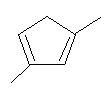$\stackrel{\mathrm{NBS}}{\to }$ Products formed are

(A)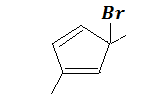(B)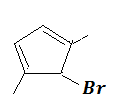(C)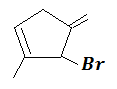(D)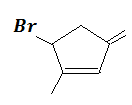1. (A) and (B) only                        2. (A) and (C) only

3. (B) and (C) only                        4. (A), (B), (C) and (D)

Concept Questions :-

Alkanes, Alkenes and Alkynes - Chemical Properties
High Yielding Test Series + Question Bank - NEET 2020

Difficulty Level:

Which reagent distinguish propyne and propene?

1. Alkaline ${\mathrm{KMnO}}_{4}$

2. ${\mathrm{Cu}}_{2}{\mathrm{Cl}}_{2}$ + ${\mathrm{NH}}_{4}\mathrm{Cl}$

3. ${\mathrm{Br}}_{2}$ water

4. ${\mathrm{Cu}}_{2}{\mathrm{Cl}}_{2}$ + ${\mathrm{NH}}_{4}\mathrm{OH}$

Concept Questions :-

Alkanes, Alkenes and Alkynes - Chemical Properties
High Yielding Test Series + Question Bank - NEET 2020

Difficulty Level:

An unsaturated hydrocarbon on complete hydrogenation gives 1-isopropyl-3 methylcyclohexane, after ozonolysis it gives one mole of formaldehyde, one mole of acetone and one mole of 2,4-Dioxohexanedial. The possible structure\s of the hydrocarbon may be

1.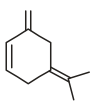2.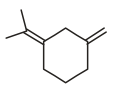3.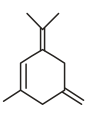4.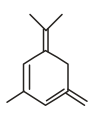Concept Questions :-

Alkanes, Alkenes and Alkynes - Chemical Properties
High Yielding Test Series + Question Bank - NEET 2020

Difficulty Level:

Which one of the following reaction is not possible?

(1) CH3COONa + HCI → CH3COOH + NaCI

(2) CH3 –SO3 H + H – C = C – Na → CH3SONa + H – C = C – H

(3) R – C = C – H + PhONa → PhOH + R – C = C – Na

(4) H – C = C – H + NaH2 → H – C = C – Na + NH3

Concept Questions :-

Alkanes, Alkenes and Alkynes - Chemical Properties